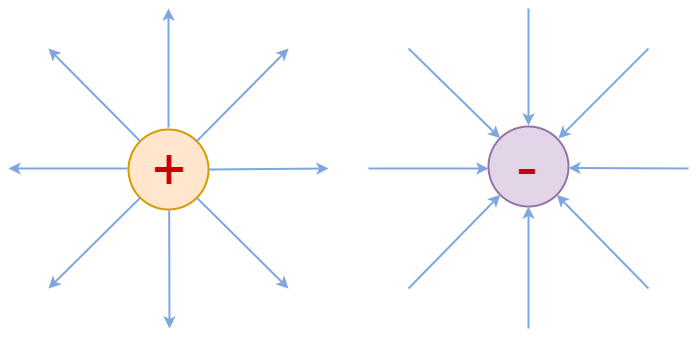GFG App
Open AppBrowser
Continue

An electric charge is surrounded by an electric field, which also exerts a force on other charges in the field. It attracts or repels them in some way. E-field is a term used to describe the electric field. Let us take a closer look at the formula for the electric field.

### What is an Electric Field?

An electric field is a region created by an electric charge that is detected when another charge is introduced into the field’s vicinity.

The electric field is a vector field that associates the (electrostatic or Coulomb) force/unit of charge exerted on an infinitesimal positive test charge at rest at each point in space.

The electric field is the area where an electric charge’s influence can be seen. When an additional charge is introduced into the field, the presence of an electric field becomes apparent. The electric field will either attract or repel the incoming charge, depending on the charge’s composition. Any electric charge has an electric field, which can be considered a property. The charge and electrical force working in the electric field define the field’s strength or intensity.

SI unit for an electric field is N/c (Newton/Coulomb)

## What is Electric Field Line?

The concept of an electric field line is used to define an electric field near charged particles. They were first used by Michael Faraday to define an electric field due to an electron and a proton.A positive charge i.e. proton sends away electric field lines whereas a negative charge i.e. an electron attracts the electric field lines. The above diagram shows the electric field due to an electron and proton separately. The electric field lines due to the system of charges are different from the electric field lines due to individual charges. Also, the density of the electric field lines shows the strength of the electric field i.e the more electric field lines in an area the more the strength of the electric field there.

## Properties of Electric Field Lines

The electric field lines have the following properties:

• Electric field lines never intersect each other.
• Electric field lines are always perpendicular to the surface of the charge.
• For a single charge, the field lines go from the surface of the charge to up to infinity.
• The magnitude of the electric field and the number of field lines, both are proportional to each other.

## Rules for Drawing Electric Field Lines

The rules used for drawing electric field lines are discussed below:

• For positive charge, electric field lines always start at their surface and go up to infinity, for negative charge it starts from infinity and ends at its surface.
• The more field lines in an area the more the strength of the electric field.
• Electric field lines never intersect each other.
• Electric field and electric field lines are always tangent at the point where they pass through.

## Solved Examples on Electric Field Lines

Example 1: At any time, a force of 10 N is operating on the charge of 18 μC. Determine the intensity of the electric field at that location.

Solution:

Given:
F = 10 N, q = 18 μC

E = F/q
= 10 / 18 × 10-6
= 5.5 × 105 N/C

Example 2: Calculate the strength of the electric field at a distance of 12 cm with a voltage of 68 V.

Solution:

Given:
V = 68 V, r = 12 cm

E = V/r

= 68/12
= 5.66 V/cm

Example 3: A force of 12 N is acting on the charge of 27 μC. Find Electric Field.

Solution:

Given:
F = 12 N, q = 27 μC

E = F/q

= 12 / 27 × 10-6
= 4.44 × 105 N/C

Example 4: The electric field is 6.4 N/C at a distance of 6 cm then find the voltage.

Solution:

Given:
E = 6.4 N/C, r = 6 cm

V = E × r

= 6.4 × 6
= 38.4 V

## FAQs on Electric Field Lines

Question 1: Write two applications for the Electric field.

Applications of Electric Field are:

• Different crystallization processes, such as nucleation, crystal development, and so on, are controlled by the electric field.
• It’s a technique for studying tissue dynamics.

Question 2: What is the SI unit of the Electric Field?

N/C (Newton/Coulomb) is the SI unit for an electric field.

Question 3: State Coulomb’s law in Electrostatics.

Coulomb’s Law states that, “Magnitude of the electrostatic force of attraction or repulsion between any two point charges is directly proportional to the product of their magnitudes, and is inversely proportional to the square of the distance between them.”

Question 4: What is an electric charge?